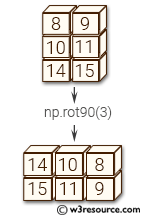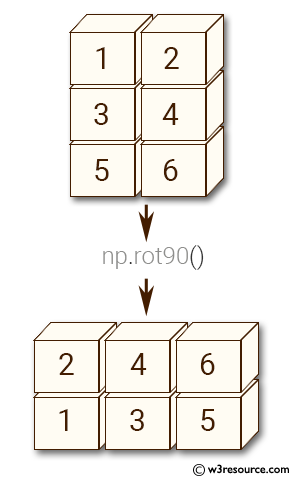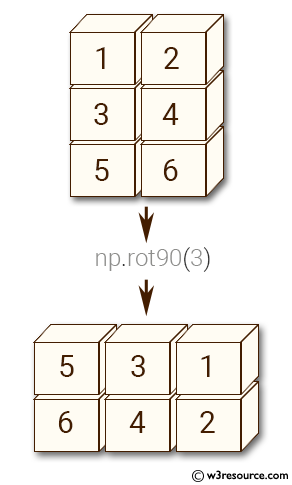# NumPy Array manipulation: rot90() function

## numpy.rot90() function

The rot90() function is used to rotate an array by 90 degrees in the plane specified by axes.
Rotation direction is from the first towards the second axis.

Syntax:

`numpy.rot90(m, k=1, axes=(0, 1))`Version: 1.15.0

Parameter:

Name Description Required /
Optional
m Array of two or more dimensions. Required
k Number of times the array is rotated by 90 degrees. Required
axes The array is rotated in the plane defined by the axes. Axes must be different. Required

Return value:

y : ndarray - A rotated view of m.

Example-1: numpy.rot90() function

``````>>> import numpy as np
>>> m = np.array([[1,2], [3,4], [5,6]], int)
>>> m
array([[1, 2],
[3, 4],
[5, 6]])
>>> np.rot90(m)
array([[2, 4, 6],
[1, 3, 5]])
``````

Pictorial Presentation:Example-2: numpy.rot90() function

``````>>> import numpy as np
>>> m = np.array([[1,2], [3,4], [5,6]], int)
>>> np.rot90(m, 3)
array([[5, 3, 1],
[6, 4, 2]])
``````

Pictorial Presentation:Python - NumPy Code Editor:

Previous: roll()
Next: NumPy Binary operation Home

﻿